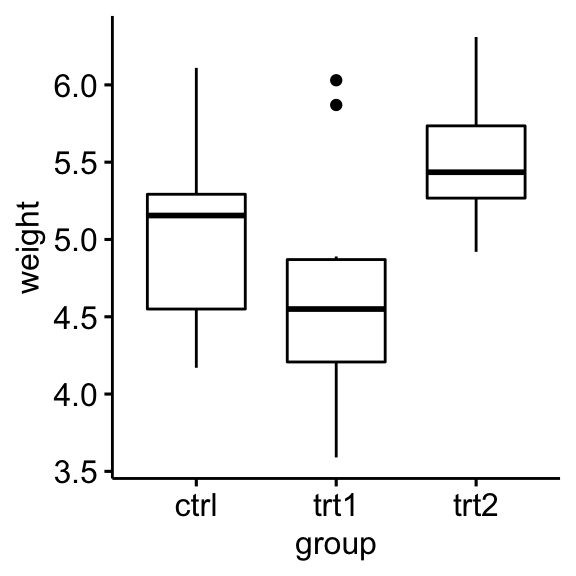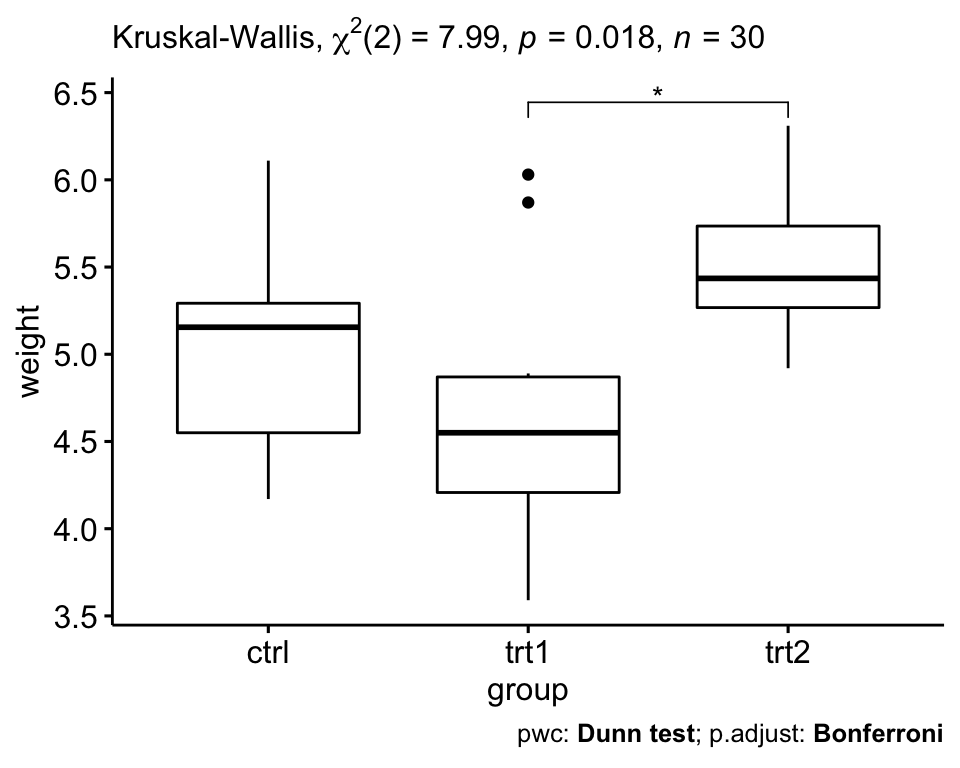# Comparing Multiple Means in R

## Kruskal-Wallis Test in R

Kruskal-Wallis test is a non-parametric alternative to the one-way ANOVA test. It extends the two-samples Wilcoxon test in the situation where there are more than two groups to compare. It’s recommended when the assumptions of one-way ANOVA test are not met.

This chapter describes how to compute the Kruskal-Wallis test using the R software. You will also learn how to calculate the effect size based on kruskal-Wallis H-statistic.

Contents:

#### Related Book

Practical Statistics in R II - Comparing Groups: Numerical Variables

## Prerequisites

Make sure you have installed the following R packages:

• tidyverse for data manipulation and visualization
• ggpubr for creating easily publication ready plots
• rstatix provides pipe-friendly R functions for easy statistical analyses.

library(tidyverse)
library(ggpubr)
library(rstatix)

## Data preparation

Here, we’ll use the built-in R data set named PlantGrowth. It contains the weight of plants obtained under a control and two different treatment conditions.

set.seed(1234)
PlantGrowth %>% sample_n_by(group, size = 1)
## # A tibble: 3 x 2
##   weight group
##    <dbl> <fct>
## 1   5.58 ctrl
## 2   6.03 trt1
## 3   4.92 trt2
• Re-order the group levels:
PlantGrowth <- PlantGrowth %>%
reorder_levels(group, order = c("ctrl", "trt1", "trt2"))

## summary statistics

Compute summary statistics by groups:

PlantGrowth %>%
group_by(group) %>%
get_summary_stats(weight, type = "common")
## # A tibble: 3 x 11
##   group variable     n   min   max median   iqr  mean    sd    se    ci
##   <fct> <chr>    <dbl> <dbl> <dbl>  <dbl> <dbl> <dbl> <dbl> <dbl> <dbl>
## 1 ctrl  weight      10  4.17  6.11   5.16 0.743  5.03 0.583 0.184 0.417
## 2 trt1  weight      10  3.59  6.03   4.55 0.662  4.66 0.794 0.251 0.568
## 3 trt2  weight      10  4.92  6.31   5.44 0.467  5.53 0.443 0.14  0.317

## Visualization

Create a box plot of weight by group:

ggboxplot(PlantGrowth, x = "group", y = "weight")## Computation

Question: We want to know if there is any significant difference between the average weights of plants in the 3 experimental conditions.

We’ll use the pipe-friendly kruskal_test() function [rstatix package], a wrapper around the R base function kruskal.test().

res.kruskal <- PlantGrowth %>% kruskal_test(weight ~ group)
res.kruskal
## # A tibble: 1 x 6
##   .y.        n statistic    df      p method
## * <chr>  <int>     <dbl> <int>  <dbl> <chr>
## 1 weight    30      7.99     2 0.0184 Kruskal-Wallis

## Effect size

The eta squared, based on the H-statistic, can be used as the measure of the Kruskal-Wallis test effect size. It is calculated as follow : eta2[H] = (H - k + 1)/(n - k); where H is the value obtained in the Kruskal-Wallis test; k is the number of groups; n is the total number of observations (M. T. Tomczak and Tomczak 2014).

The eta-squared estimate assumes values from 0 to 1 and multiplied by 100 indicates the percentage of variance in the dependent variable explained by the independent variable.

The interpretation values commonly in published literature are: 0.01- < 0.06 (small effect), 0.06 - < 0.14 (moderate effect) and >= 0.14 (large effect).

PlantGrowth %>% kruskal_effsize(weight ~ group)
## # A tibble: 1 x 5
##   .y.        n effsize method  magnitude
## * <chr>  <int>   <dbl> <chr>   <ord>
## 1 weight    30   0.222 eta2[H] large

A large effect size is detected, eta2[H] = 0.22.

## Multiple pairwise-comparisons

From the output of the Kruskal-Wallis test, we know that there is a significant difference between groups, but we don’t know which pairs of groups are different.

A significant Kruskal-Wallis test is generally followed up by Dunn’s test to identify which groups are different. It’s also possible to use the Wilcoxon’s test to calculate pairwise comparisons between group levels with corrections for multiple testing.

Compared to the Wilcoxon’s test, the Dunn’s test takes into account the rankings used by the Kruskal-Wallis test. It also does ties adjustments.

• Pairwise comparisons using Dunn’s test:
# Pairwise comparisons
pwc <- PlantGrowth %>%
dunn_test(weight ~ group, p.adjust.method = "bonferroni")
pwc
## # A tibble: 3 x 9
## * <chr>  <chr>  <chr>  <int> <int>     <dbl>   <dbl>  <dbl> <chr>
## 1 weight ctrl   trt1      10    10     -1.12 0.264   0.791  ns
## 2 weight ctrl   trt2      10    10      1.69 0.0912  0.273  ns
## 3 weight trt1   trt2      10    10      2.81 0.00500 0.0150 *
• Pairwise comparisons using Wilcoxon’s test:
pwc2 <- PlantGrowth %>%
wilcox_test(weight ~ group, p.adjust.method = "bonferroni")
pwc2
## # A tibble: 3 x 9
## * <chr>  <chr>  <chr>  <int> <int>     <dbl> <dbl> <dbl> <chr>
## 1 weight ctrl   trt1      10    10      67.5 0.199 0.597 ns
## 2 weight ctrl   trt2      10    10      25   0.063 0.189 ns
## 3 weight trt1   trt2      10    10      16   0.009 0.027 *

The pairwise comparison shows that, only trt1 and trt2 are significantly different (Wilcoxon’s test, p = 0.027).

## Report

There was a statistically significant differences between treatment groups as assessed using the Kruskal-Wallis test (p = 0.018). Pairwise Wilcoxon test between groups showed that only the difference between trt1 and trt2 group was significant (Wilcoxon’s test, p = 0.027)

# Visualization: box plots with p-values
pwc <- pwc %>% add_xy_position(x = "group")
ggboxplot(PlantGrowth, x = "group", y = "weight") +
stat_pvalue_manual(pwc, hide.ns = TRUE) +
labs(
subtitle = get_test_label(res.kruskal, detailed = TRUE),
caption = get_pwc_label(pwc)
)